# Supergravity calculation

Hi everyone, here I tried to make some supergravity calculations for N=1, D=4. I needed to use the "substitute" command a lot. Any suggestions to make it more neat and tidy are very welcome. Also is there any other way than using the substitute command to make the cubic expressions vanish?

D{#}::Derivative;
\bar{D{#}}::Derivative;
\bar{#}::DiracBar;
{m,n,p,q,r,s,t,u,m#}::Indices(flat);
{m,n,p,q,r,s,t,u,m#}::Integer(0..3);
{\mu,\nu,\rho,\sigma,\kappa,\lambda,\alpha,\beta}::Indices(curved,position=fixed);
{\mu,\nu,\rho,\sigma,\kappa,\lambda,\alpha,\beta}::Integer(0..3);

e^{m \mu}::Vielbein;
e^{m}_{\mu}::Vielbein;
e_{m \mu}::InverseVielbein;
e_{m}^{\mu}::InverseVielbein;
e::Determinant(e_{m \mu});
e::Determinant(e_{m}^{\mu});
e::Determinant(e^{m}_{\mu});
e::Determinant(e^{m \mu});
g^{\mu\nu}::InverseMetric;
g_{\mu\nu}::Metric;
R_{\mu \nu \rho \sigma}::RiemannTensor;

{ \epsilon,\psi_{\mu} }::Spinor(dimension=4, type=Majorana);
\Gamma_{#{m}}::GammaMatrix(metric=\delta);
{ \psi_{\mu},\epsilon }::AntiCommuting;
{ \psi_{\mu}}::SelfAntiCommuting;
{ \epsilon, \psi_{\mu}}::SortOrder;
\Gamma_{#}::Depends(\bar{#});
{ \psi_{\mu}, \epsilon ,\bar{\epsilon}}::Depends(D{#});


First, we write our action as two parts:

S32:=  \int{- 1/2 e \bar{\psi_\mu} \Gamma^{\mu\nu\rho} D_{\nu}{\psi_{\rho}}}{x};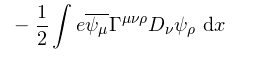S2:= \int{ 1/2 e e_{m}^{\mu} e_{n}^{\nu} R_{\mu \nu}^{ m n}}{x};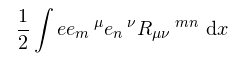Now we define our SUSY rules. (I did not include the variation of $e_{m}^{\mu}$, because it only leads to cubic expressions (for the action S32) and they vanish.)

susy32:= {e -> 1/2 e {\bar{\epsilon} \Gamma^\sigma \psi_\sigma} , \psi_\mu -> D_{\mu}{\epsilon}};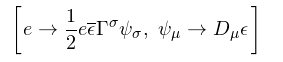susy2:= {e -> 1/2 e {\bar{\epsilon} \Gamma^\sigma \psi_\sigma}, e_{m}^{\mu} -> -1/2 {\bar{\epsilon}} \Gamma^{\mu} \psi_m };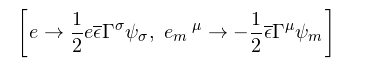Let us start to our calculations with the S32 part. First we take the
variation of it, according to the rules we defined above.

vS32= vary(S32, susy32);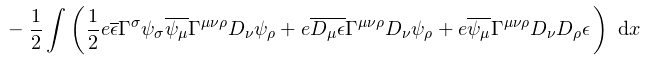Here, I need to erase the bar above the $D_{\mu}$ term because the
integrate by parts command does not work properly with the bar.

substitute(_,$\bar{D_{#??}{\epsilon}} -> D_{#??}{\bar{\epsilon}}$);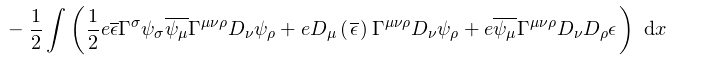The first term here, is a cubic expression therefore it must be
vanish.

substitute(_, $\bar{\epsilon}*A?? -> 0$);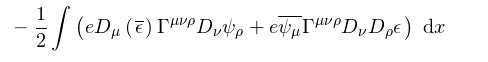Manipulating the equations a little bit leads us to use "Clifford
algebra".

integrate_by_parts(_, $\bar{\epsilon}$);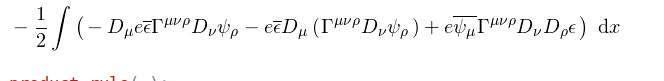product_rule(_);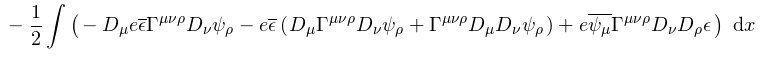We know that
$D{\mu} e = 0$
and $D {\mu}{\Gamma^{\mu\nu\rho}} = 0$ so
we substitute it by hand.

substitute(_,$D_{#??}{e}*A?? -> 0$);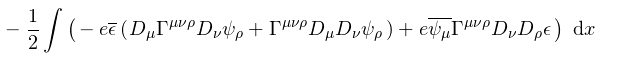substitute(_, $D_{#??}{\Gamma^{\mu\nu\rho}}*A?? -> 0$);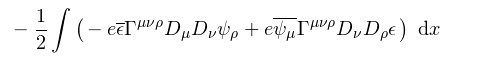Using the Clifford algebra, we can substitute the equations below;

substitute(_, $D_{\mu}{D_{\nu}{A??} } -> 1/8 R_{\mu \nu m n} \Gamma^{m n} A??$ )
sort_spinors(_)
rename_dummies(_);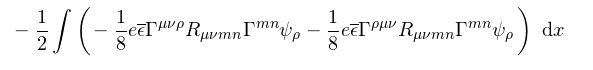substitute(_, $\Gamma^{\mu \nu \rho}R_{\mu \nu m n}\Gamma^{m n} -> 4 \Gamma^{\mu}R_{\mu \nu}^{\rho \nu} + 2 \Gamma^{\rho} R_{\mu \nu}^{\nu \mu}$);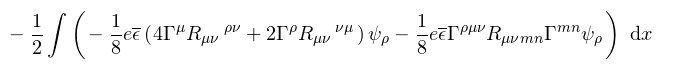substitute(_, $\Gamma^{\rho \mu \nu}R_{\mu \nu m n}\Gamma^{m n} -> 4 \Gamma^{\mu}R_{\mu \nu}^{\rho \nu} + 2 \Gamma^{\rho} R_{\mu \nu}^{\nu \mu}$);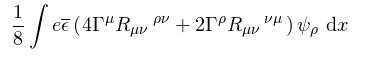Now, we can start to play with the S2 part of the action. Here we will
take the variation and re-organize the result as shown below:

vS2= vary(S2, susy2);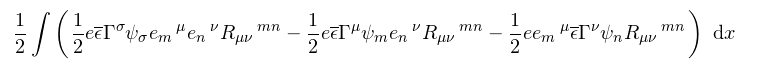eliminate_vielbein(_, repeat=True)
rename_dummies(_);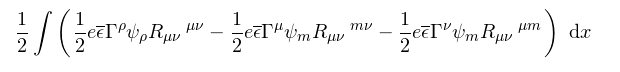We now need to use a property of the Riemann tensor such as below;

substitute(_, $R_{\mu \nu}^{\mu m} -> R_{\nu \mu}^{m \mu}$);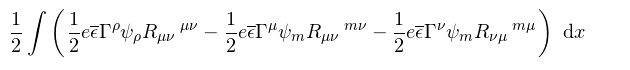canonicalise(_);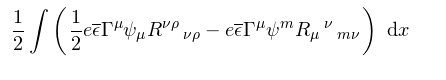Stotal=vS32+vS2;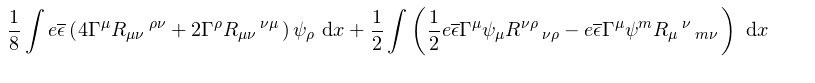This final sum must be equal to $0$, yet I couldn't accomplish to sum
the integrals to show it with cadabra.

Thanks.